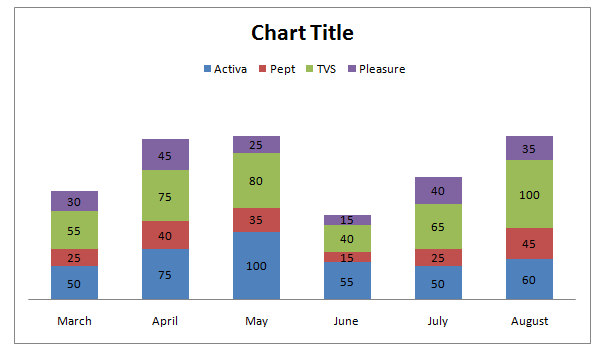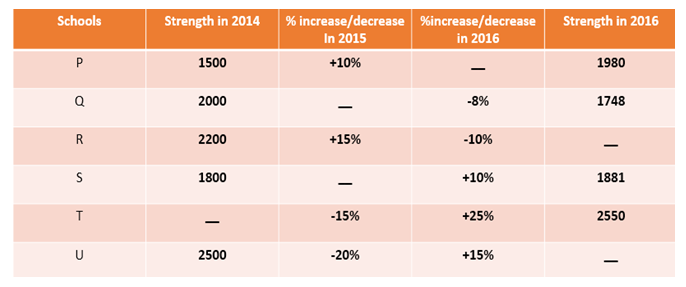# Quantitative Aptitude Questions (Data Interpretation) for SBI Clerk 2018 Day-34

## Quantitative Aptitude Questions (Data Interpretation) for SBI Clerk 2018 Day-34:

Dear Readers, SBI is conducting Online preliminary Examination for the recruitment of Clerical Cadre. preliminary Examination of SBI Clerk was scheduled from June/July 2018. To enrich your preparation here we have providing new series of Data Interpretation – Quantitative Aptitude Questions. Candidates those who are appearing in SBI Clerk Prelims Exam can practice these Quantitative Aptitude average questions daily and make your preparation effective.

Click “Start Quiz” to attend these Questions and view Solutions

## Daily Practice Test Schedule | Good Luck

 Topic Daily Publishing Time Daily News Papers & Editorials 8.00 AM Current Affairs Quiz 9.00 AM Logical Reasoning 10.00 AM Quantitative Aptitude “20-20” 11.00 AM Vocabulary (Based on The Hindu) 12.00 PM Static GK Quiz 1.00 PM English Language “20-20” 2.00 PM Banking Awareness Quiz 3.00 PM Reasoning Puzzles & Seating 4.00 PM Daily Current Affairs Updates 5.00 PM Data Interpretation / Application Sums (Topic Wise) 6.00 PM Reasoning Ability “20-20” 7.00 PM English Language (New Pattern Questions) 8.00 PM General / Financial Awareness Quiz 9.00 PM

Directions (Q. 1-5) Read the information carefully and answer the following questions.

Monthly sales turnover (in lakhs) of Various scooters like Activa, Pept, TVS and Pleasure  from March 2017 to August 2017.1. In which of the following months, the sales turnover of Activa as a percentage of the combined sales turnover of pept and TVS is least?
1. August
2. May
3. June
4. March
5. April
1. In which of the following month, Pleasure’s profit is highest, if it makes 25% profit on its sales turnover on each month?
1. August
2. May
3. April
4. July
5. June
1. Total sales turn over in the month of march and april is what percentage more than the total sales turn over in the month of july?
1. 67 %
2. 44 %
3. 17 %
4. 104.32 %
5. None of these
1. In how many months, the sales turnover of Activa was more than the one third of the total sales turnover of the four companies?
1. One
2. Three
3. Four
4. Two
5. Five
1. The average no of sales turn over in the month of June, July and August?
1. 176.34
2. 167.89
3. 181.67
4. 153.89
5. None of these

Directions (Q. 6 – 10) Study the following information carefully and answer the questions given below:

The following table represents strength of six different school in 2014 and 2016 and percentage increase/decrease in 2015 and 2016. Some data are missing.1. Find the sum of strength of school U in 2015 and the strength of school R in 2016.
1. 3456
2. 2389
3. 4277
4. 4215
5. none of these
1. Percentage increase/decrease in the strength of school P in 2016 is what percent of the percentage increase/decrease in the strength of school Q in 2015?
1. 450%
2. 400%
3. 320%
4. 250%
5. none of these
1. Strength of school T in 2014 is what percent more than the strength of school Q in 2014?
1. 20%
2. 14%
3. 15%
4. 22 %
5. none of these
1. Find the sum of strength of all the schools in 2016.
1. 13245
2. 12736
3. 11637
4. 14235
5. none of these
1. Find the respective ratio of the percentage increase/decrease in the strength of S in 2015 and that of P in 2016.
1. 4:1
2. 2:3
3. 1:4
4. 3:2
5. none of these

Directions (Q. 1-5)

Required percentage =Activa / (Pept+TVS) X 100

In March=50/( 25+55) X 100=62.5%

April=75/(40+75) X 100=65.2%

May=100/(35+80) X 100=87%

June=55/(15+40) X 100=100%

July= 50/(25+65) X 100=55.5%

August=60/(45+100) X 100=41.4%

From this, we observe that 41.4% is the least percentage.

Monthly’s turnover of Pleasure:

March=30 lakhs

Then profit =  (30 X 25)/100=7.5 lakhs

April=45 lakhs

Then profit =  (45 X 25)/100=11.25lakhs

May=25 lakhs

Then profit =  (25 X 25)/100=6.25 lakhs

June=15 lakhs

Then profit =  (15 X 25)/100=3.75 lakhs

July=40 lakhs

Then profit =  (40 X 25)/100=10 lakhs

August=35 lakhs

Then profit =  (35 X 25)/100=8.75 Lakhs

Thus the highest profit is in the April month.

Total sales turn over in the month of march and april

= > 160 + 235 = 395

Total sales turn over in the month of july

= > 180

Required % = ((395 – 180) / 180)*100 = 119.44 %

March=50/160=5/16<1/3

April=75/235=15/47<1/3

May=100/240=10/(24 )>1/3

June=55/125=11/25>1/3

July=50/180=5/18<1/3

August=60/240=1/4<1/3

Only 2 months.

Total sales turn over in the month of June, July and August

= > 125 + 180 + 240

Average = 545/3 = 181.67

Directions (Q. 6 – 10)

Strength of school U in 2015 = 2500 x 80/100 = 2000

Strength of school R in 2016 = 2200 x 115/100 x 90/100 = 2277

Required sum = 2000 + 2277 = 4277

Let percentage increase/decrease in the strength of school P in 2016 = x%

And percentage increase/decrease in the strength of school Q in 2015 = y%

Now,

1500 x 110/100 x (100 + x)/100 = 1980

=> (100 + x)/100 = 1980 x 100/110 x 1/1500

=> (100 + x)/100 = 1.2

=> 100 + x = 120

=> x = 20 => 20% increase

And

2000 x (100 + y)/100 x 92/100 = 1748

=>(100 + y)/100 = 1748 x 100/92 x 1/2000

=> (100 + y)/100 = 0.95

=> 100 + y = 95

=> y = -5 => 5% decrease

Required percentage = (20/5) x 100 = 400%

Let the strength of school T in 2014 = y

y x 85/100 x 125/100 = 2550

=> y = 2550 x 100/125 x 100/85

=> y = 2400

Required percentage = {(2400 – 2000)/2000} x 100 = 400/2000 x 100 = 20%

Strength of school R in 2016 = 2200 x 115/100 x 90/100 = 2277

Strength of school U in 2016 = 2500 x 80/100 x 115/100 = 2300

Required sum = 1980 + 1748 + 2277 + 1881 + 2550 + 2300 = 12736

Let percentage increase/decrease in the strength of school S in 2015 = x%

And percentage increase/decrease in the strength of school P in 2016 = y%

1800 x (100 + x)/100 x 110/100 = 1881

=> (100 + x)/100 = 1881 x 100/110 x 1/1800

=> (100 + x)/100 = 0.95

=> 100 + x = 95

=> x = -5 => 5% decrease

1500 x 110/100 x (100 + y)/100 = 1980

=> (100 + y)/100 = 1980 x 100/110 x 1/1500

=> (100 + y)/100 = 1.2

=> 100 + y = 120

=> y = 20 => 20% increase

Required ratio = 5:20 = 1:4